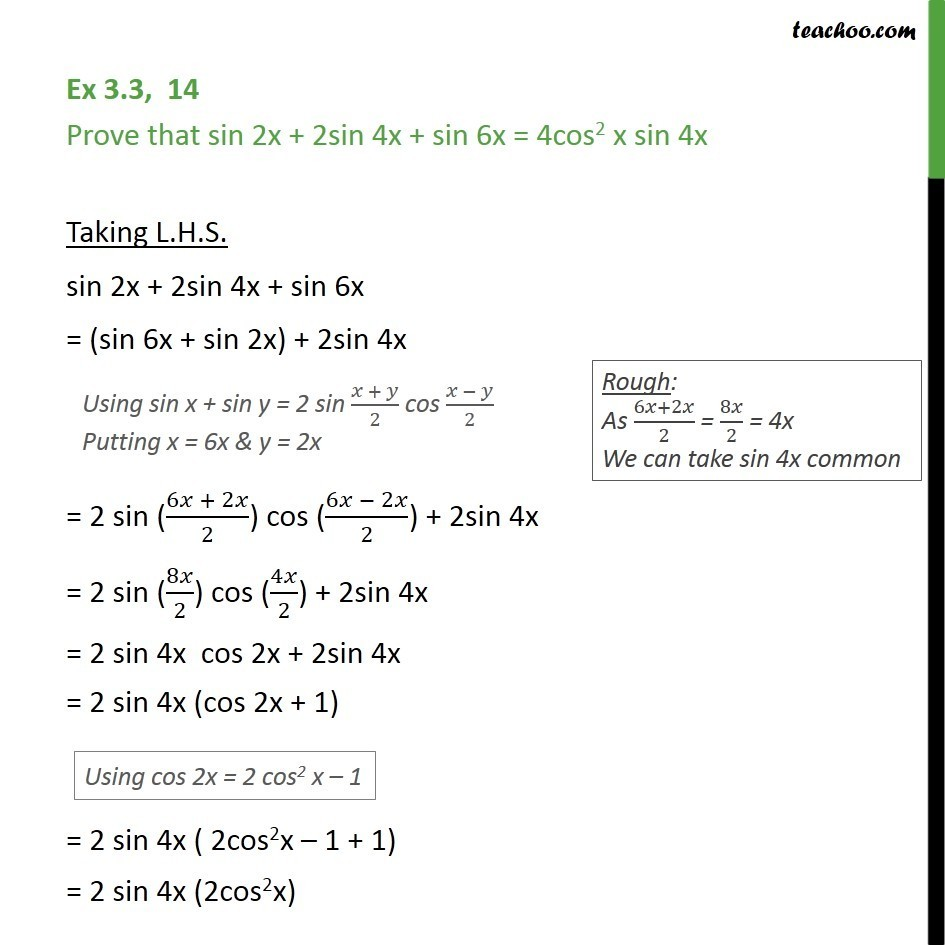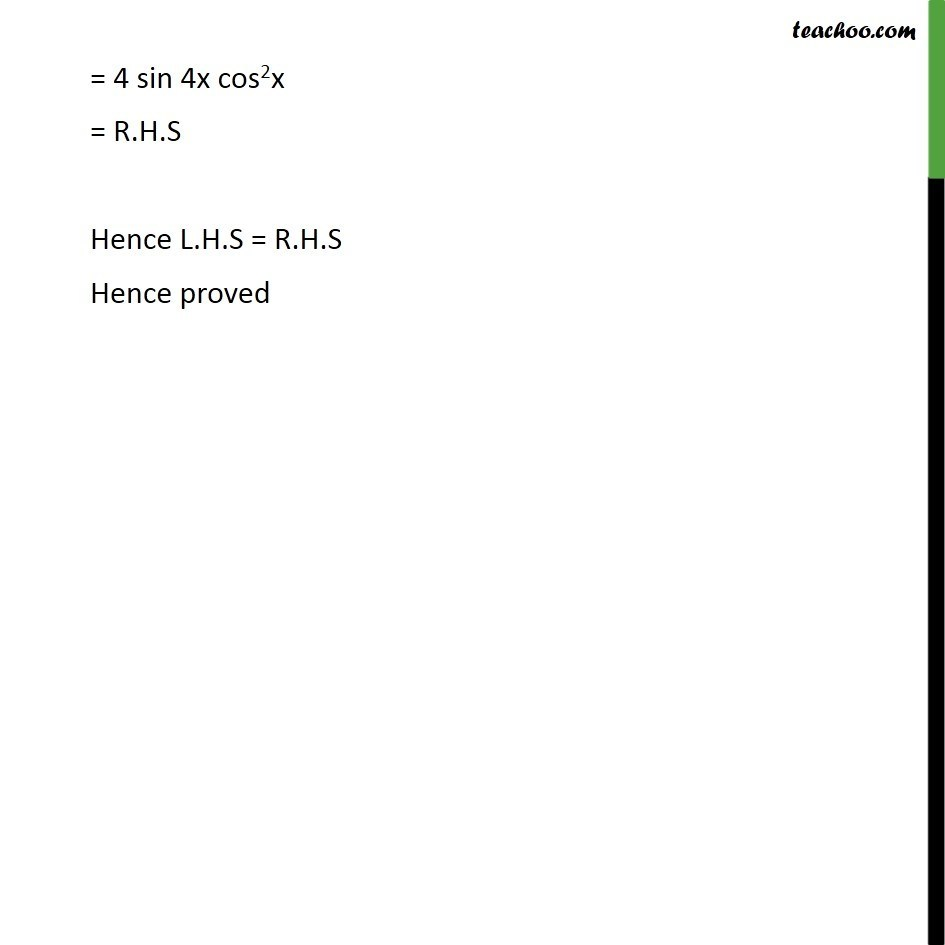Subscribe to our Youtube Channel - https://you.tube/teachoo

1. Chapter 3 Class 11 Trigonometric Functions
2. Concept wise
3. Cos x + cos y formula

Transcript

Ex 3.3, 14 Prove that sin 2x + 2sin 4x + sin 6x = 4cos2 x sin 4x Taking L.H.S. sin 2x + 2sin 4x + sin 6x = (sin 6x + sin 2x) + 2sin 4x = 2 sin ((6 + 2 )/2) cos ((6 2 )/2) + 2sin 4x = 2 sin (8 /2) cos (4 /2) + 2sin 4x = 2 sin 4x cos 2x + 2sin 4x = 2 sin 4x (cos 2x + 1) = 2 sin 4x ( 2cos2x 1 + 1) = 2 sin 4x (2cos2x) = 4 sin 4x cos2x = R.H.S Hence L.H.S = R.H.S Hence proved

Cos x + cos y formula